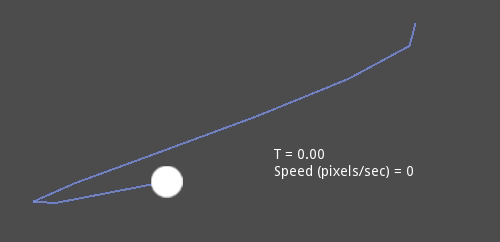# 贝塞尔，曲线和路径¶

## 二次贝塞尔曲线¶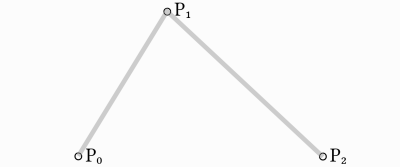```func _quadratic_bezier(p0: Vector2, p1: Vector2, p2: Vector2, t: float):
var q0 = p0.linear_interpolate(p1, t)
var q1 = p1.linear_interpolate(p2, t)
```

```var r = q0.linear_interpolate(q1, t)
return r
```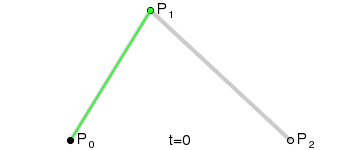（图像来源：维基百科）

## 三次贝塞尔曲线¶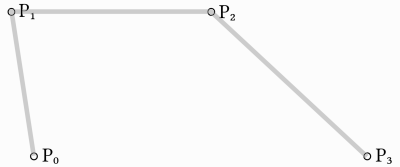```func _cubic_bezier(p0: Vector2, p1: Vector2, p2: Vector2, p3: Vector2, t: float):
```

```var q0 = p0.linear_interpolate(p1, t)
var q1 = p1.linear_interpolate(p2, t)
var q2 = p2.linear_interpolate(p3, t)
```

```var r0 = q0.linear_interpolate(q1, t)
var r1 = q1.linear_interpolate(q2, t)
```

And to one:

```var s = r0.linear_interpolate(r1, t)
return s
```

Here is the full function:

```func _cubic_bezier(p0: Vector2, p1: Vector2, p2: Vector2, p3: Vector2, t: float):
var q0 = p0.linear_interpolate(p1, t)
var q1 = p1.linear_interpolate(p2, t)
var q2 = p2.linear_interpolate(p3, t)

var r0 = q0.linear_interpolate(q1, t)
var r1 = q1.linear_interpolate(q2, t)

var s = r0.linear_interpolate(r1, t)
return s
```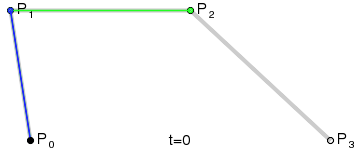（图像来源：维基百科）

• “point0 = p0”:是第一个点，即源
• “control0 = p1 - p0”：是相对于第一个控制点的向量
• “control1 = p3 - p2”：是相对于第二个控制点的向量
• “point1 = p3”：是第二个点，即终点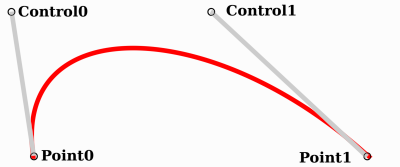## 二维曲线，三维曲线，路径和二维路径¶

There are two objects that contain curves: Curve3D and Curve2D (for 3D and 2D respectively).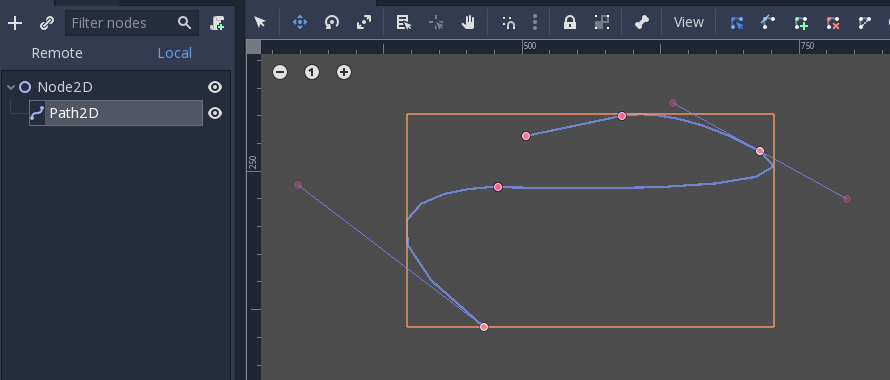## 评估¶

```var t = 0.0

func _process(delta):
t += delta
position = _cubic_bezier(p0, p1, p2, p3, t)
```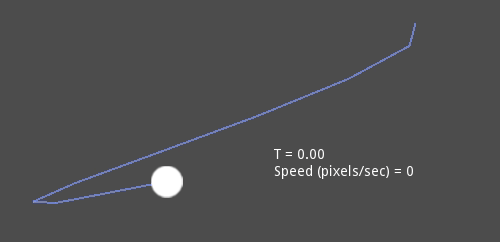## 绘制¶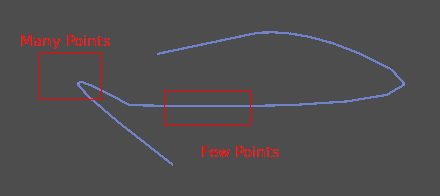Before drawing Bezier curves, tessellation is required. This is often done with a recursive or divide and conquer function that splits the curve until the curvature amount becomes less than a certain threshold.

*曲线*类通过:ref:`二维曲线.细分曲面() <class_Curve2D_method_tessellate>`提供了这个特性。（其接收可选的递归“阶段”和角度“公差”参数）。这样，根据曲线画东西就容易多了。

## 遍历¶

```var t = 0.0

func _process(delta):
t += delta
position = curve.interpolate_baked(t * curve.get_baked_length(), true)
```